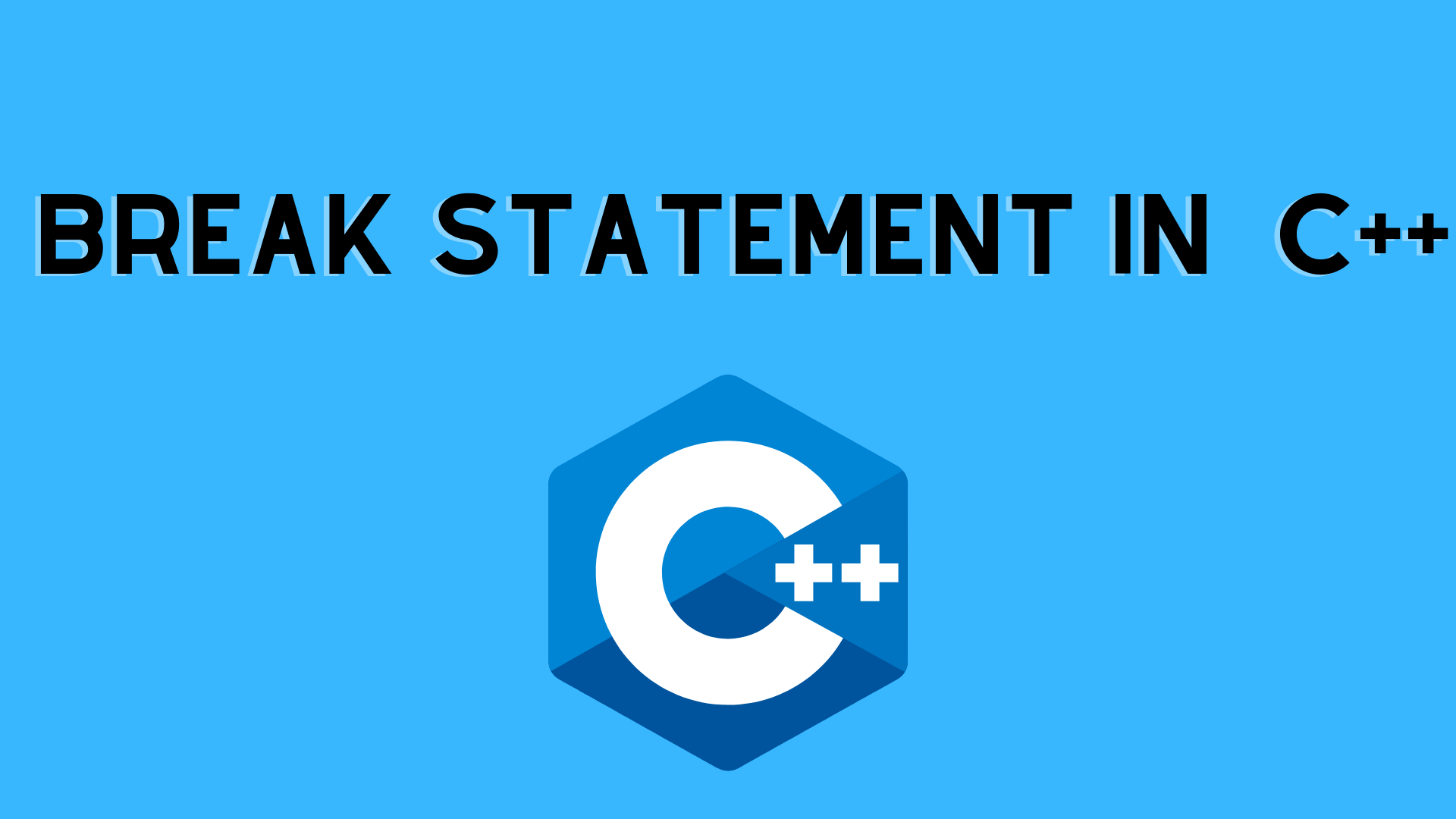## C++ Break Statement with Example | C++ Programming

In this tutorial, we will learn about the break statement & its working in loops with the help of examples.

## What is break statement?

break statement is a loop control statement that is used to end a loop. When the break statement is encountered within a loop, the loop iterations stop and control returns from the loop to the first statement after the loop.

• The break statement in C++ immediately terminates the loop, and control of the program moves to the statement following the loop.
• It is almost always used with decision-making statements (C++ if- else Statement).

The break statement in C++ has the following syntax:

Syntax:

–> Break statements are generally used when we are unsure of the number of iterations for a loop or when we want to terminate the loop based on some condition.

Example: Break statement

```#include <iostream>
using namespace std;

int main() {
for(int i=1;i<=10;i++){
if(i==4){
//break statement
break;
}
cout<<i<<endl;
}
return 0;
}  ```

Output

Example: Break With Inner Loop:

```#include <iostream>
using namespace std;
int main()
{
for(int i=1;i<=3;i++){
for(int j=1;j<=3;j++){
if(i==2&&j==2){
break;
}
cout<<i<<" "<<j<<"\n";
}
}
}  ```

Output

1 1
1 2
1 3
2 1
3 1
3 2
3 3

Example: Break with for loop:

```// program to print the value of i

#include <iostream>
using namespace std;

int main() {
for (int i = 1; i <= 5; i++) {
// break condition
if (i == 3) {
break;
}
cout << i << endl;
}

return 0;
}```

Output

1
2

This indicates that the break statement ends the loop when I equal to 3. As a result, no values larger than or equal to 3 are included in the output.

Example: Break In while loop

```#include <iostream>
using namespace std;

int main() {
int number;
int sum = 0;

while (true) {
// take input from the user
cout << "Enter a number: ";
cin >> number;

// break condition
if (number < 0) {
break;
}

sum += number;
}

// display the sum
cout << "The sum is " << sum << endl;

return 0;
}```

Output

Enter a number: 1
Enter a number: 2
Enter a number: 3
Enter a number: -5
The sum is 6.

• The user enters a number in the above software. The while loop is used to print the total sum of the user’s numbers. Take note of the code here:
• This means that if the user enters a negative value, the break statements stops the loop and executes the code outside of it.
• The while loop will keep running until the user submits a negative number.

Example: Break with Nested loop

```#include <iostream>
using namespace std;

int main() {
int number;
int sum = 0;

// nested for loops

// first loop
for (int i = 1; i <= 3; i++) {
// second loop
for (int j = 1; j <= 3; j++) {
if (i == 2) {
break;
}
cout << "i = " << i << ", j = " << j << endl;
}
}

return 0;
}```

Output

i = 1, j = 1
i = 1, j = 2
i = 1, j = 3
i = 3, j = 1
i = 3, j = 2
i = 3, j = 3

Example: Break statements in switch case

```#include <stdio.h>
int main()
{
int x = 3;
switch (x) {
case 1:
printf("Choice is 1");
break;
case 2:
printf("Choice is 2");
break;
case 3:
printf("Choice is 3");
break;
default:
printf("Choice other than 1, 2 and 3");
break;
}
return 0;
}
```

Output

Choice is 3

You may like:

C++ switch case statement with Example | C++ Programming

While and do…while loop with Example | C++ Programming